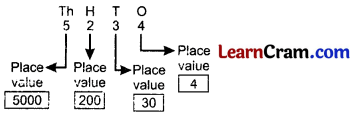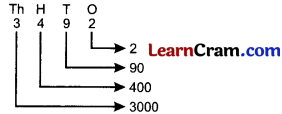# DAV Class 3 Maths Chapter 1 Worksheet 4 Solutions

The DAV Class 3 Maths Book Solutions and DAV Class 3 Maths Chapter 1 Worksheet 4 Solutions of Numbers upto 9999 offer comprehensive answers to textbook questions.

## DAV Class 3 Maths Ch 1 WS 4 Solutions

Question 1.
Draw the abacus in your notebook and show the value of the encircled digit. Also write the value.

(a) 5 84
Solution:
5 84Place value of 2 = 20 (2 tens)

(b) 9 0 8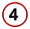Solution:
9 0 8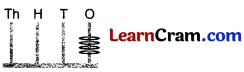Place value of 4 = 4 (4 ones)(c)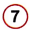2 5 3
Solution:2 5 3Place value of 7 = 7000

(d) 6 6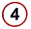2
Solution:
6 62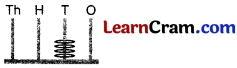Place value of 4 = 4 tens = 40

(e) 1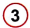2 4
Solution:
12 4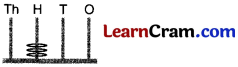Place value of 3 = 3 hundred = 300

(f) 6 88
Solution:
6 88Place value of 0 = 0 Tens = 0Question 2.
Write the value of the encircled digit in the given numbers.

(a)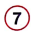7 9 6
Solution:7 9 6

Value = 7000

(b) 3 4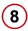0
Solution:
3 40

Value = 80

(c) 1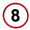9 6
Solution:
19 6

Value = 800

(d) 8 9 4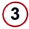Solution:
8 9 4Value = 3

(e) 2 1 6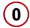Solution:
2 1 6Value = 0

(f) 2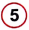0 3
Solution:
20 3

Value = 500### DAV Class 3 Maths Chapter 1 Worksheet 4 Notes

The position of a digit in a number gives its place value.

Example: